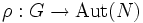# Characteristic-semidirectly extensible automorphism

## Contents

BEWARE! This term is nonstandard and is being used locally within the wiki. [SHOW MORE]
This article defines an automorphism property, viz a property of group automorphisms. Hence, it also defines a function property (property of functions from a group to itself)
View other automorphism properties OR View other function properties
This is a variation of extensible automorphism|Find other variations of extensible automorphism |
This term is related to: Extensible automorphisms problem
View other terms related to Extensible automorphisms problem | View facts related to Extensible automorphisms problem

## Definition

### Definition with symbols

Let$\sigma$ be an automorphism of a group$G$. We say that$\sigma$ is characteristic-semidirectly extensible or a CSE-automorphism if the following holds:

Let$\rho:G \to \operatorname{Aut}(N)$ be a homomorphism such that$N$ is a characteristic subgroup of the associated semidirect product$M = N \rtimes G$. Then, there exists an automorphism$\phi$ of$N$ whose restriction to$G$ is$\sigma$.

## Relation with other proeprties

### Weaker properties

For a finite group, linearly pushforwardable automorphism over a prime field where the prime does not divide the order of the group. For full proof, refer: finite-characteristic-semidirectly extensible implies linearly pushforwardable over prime field